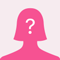# I need homework help.... please..?The Number of apples produced by each tree in an apple orchard depends on how densely the trees are planted. If and trees are planted on an acre of land, then each tree produces 900 – 9n apples. So the number of apples produced per acre is A(n) = n(900-9n).

How many trees should be planted per acre in order to obtain the maximum yield of apples?

and

A manufacturer finds that the revenue generated by selling x units of a certain commodity is given by the function R(x) = 80x - .5x^2, where the revenue R(x) is measured in dollars.

What is the maximum revenue?

How many units should be manufactured to obtain this maximum?

I don't want you to just answer I would like you to explain it to me.